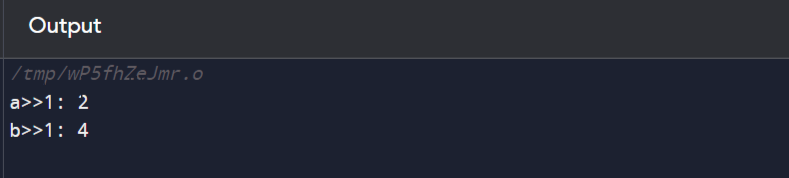# C++教程-C++位操作## C++位操作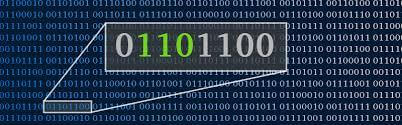### 位

1 - 表示信号存在或为真

0 - 表示信号不存在或为假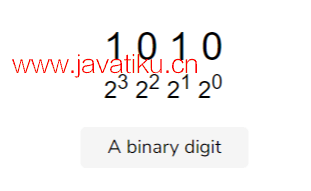### 位操作

• 逻辑与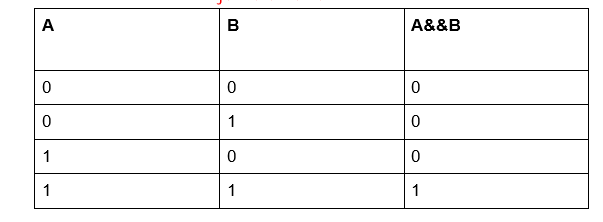C++程序

``````#include <iostream>
using namespace std;

int main() {
int a = 5;
int b = 9;

// false && false = false
cout << ((a == 0) && (a > b)) << endl;

// false && true = false
cout << ((a == 0) && (a < b)) << endl;

// true && false = false
cout << ((a == 5) && (a > b)) << endl;

// true && true = true
cout << ((a == 5) && (a < b)) << endl;

return 0;
}  ``````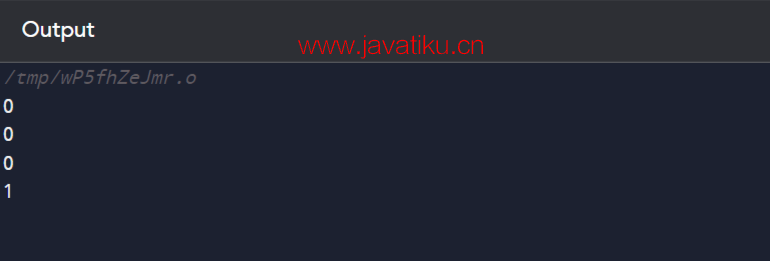• 逻辑或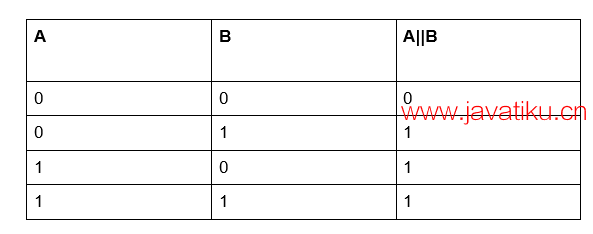C++程序

``````#include <iostream>
using namespace std;

int main() {
int a = 5;
int b = 9;

// false && false = false
cout << ((a == 0) || (a > b)) << endl;

// false && true = true
cout << ((a == 0) || (a < b)) << endl;

// true && false = true
cout << ((a == 5) || (a > b)) << endl;

// true && true = true
cout << ((a == 5) || (a < b)) << endl;

return 0;
}``````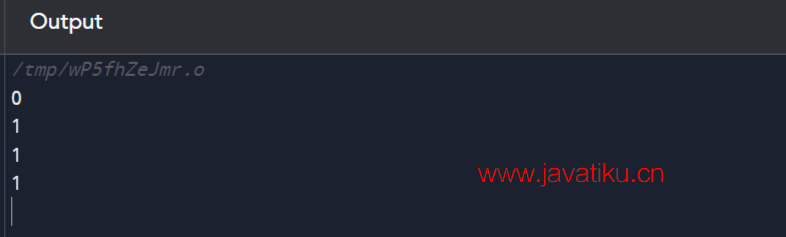• 逻辑非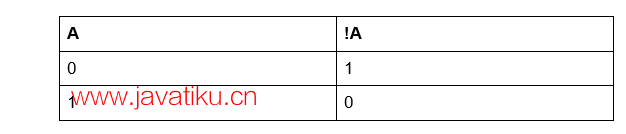C++程序

``````#include <iostream>
using namespace std;

int main() {
int a = 5;

// !false = true
cout << !(a == 0) << endl;

// !true = false
cout << !(a == 5) << endl;

return 0;
}``````• 左移运算符

C++程序

``````#include<iostream>
using namespace std;
int main()
{
// a = 5(00000101), b = 9(00001001)
unsigned char a = 5, b = 9;

// 结果为00001010
cout << "a<<1: "<<  (a<<1) << "\n";

// 结果为00010010
cout << "b<<1: " <<  (b<<1);
return 0;
}``````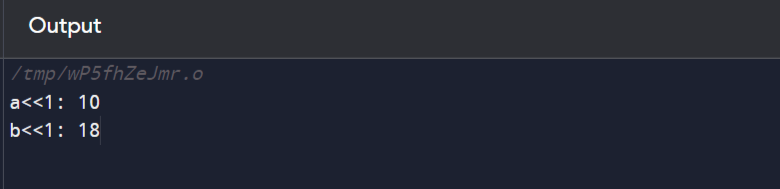• 右移运算符

C++程序

``````#include <bits/stdc++.h>
using namespace std;
int main()
{
// a = 5(00000101), b = 9(00001001)
unsigned char a = 5, b = 9;

// 结果为00000010

cout<< "a>>1: " <<  (a >> 1) << "\n";

// 结果为00000100
cout<< "b>>1: " <<  (b >> 1);
return 0;
}``````